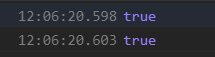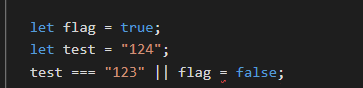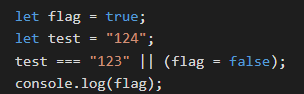Solo  当前访客：3

# 海西看月亮🌕

## JavaScript 表达式与赋值 小思考

2019-08-20 23:04:09

``````       let flag = true;
let test = "123";
let a =  test === "123" || (flag = false);
//这里如果括号去除，会报错
console.log(a)
console.log(flag)
````````````      let flag = false;
let test = "123";
let a =  (flag = true);
console.log(a)
console.log(flag);
``````TOP